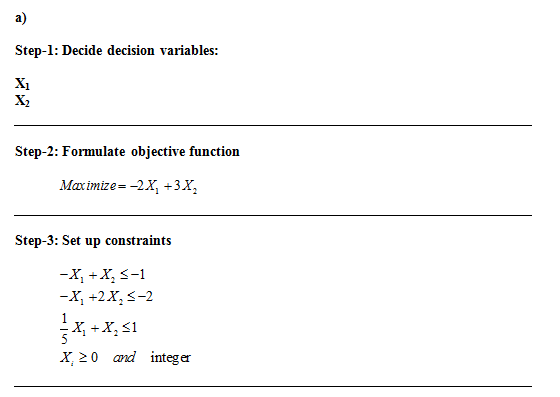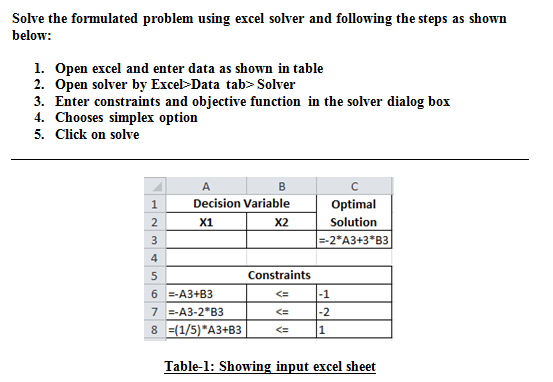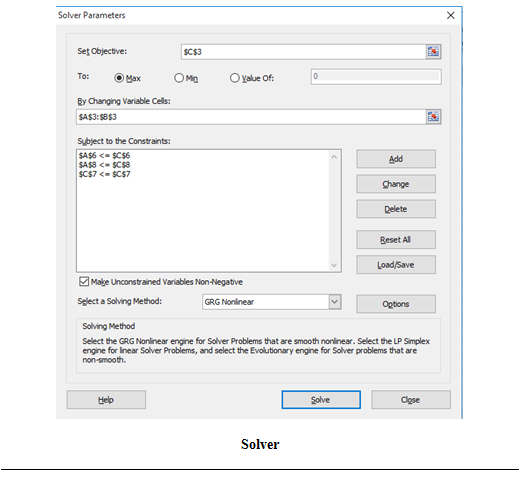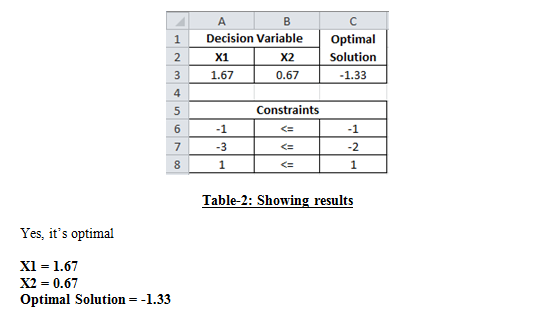# Answered! Lets revisit exam problem 2 but now add the condition that the decision variables are integers, that is: Objective…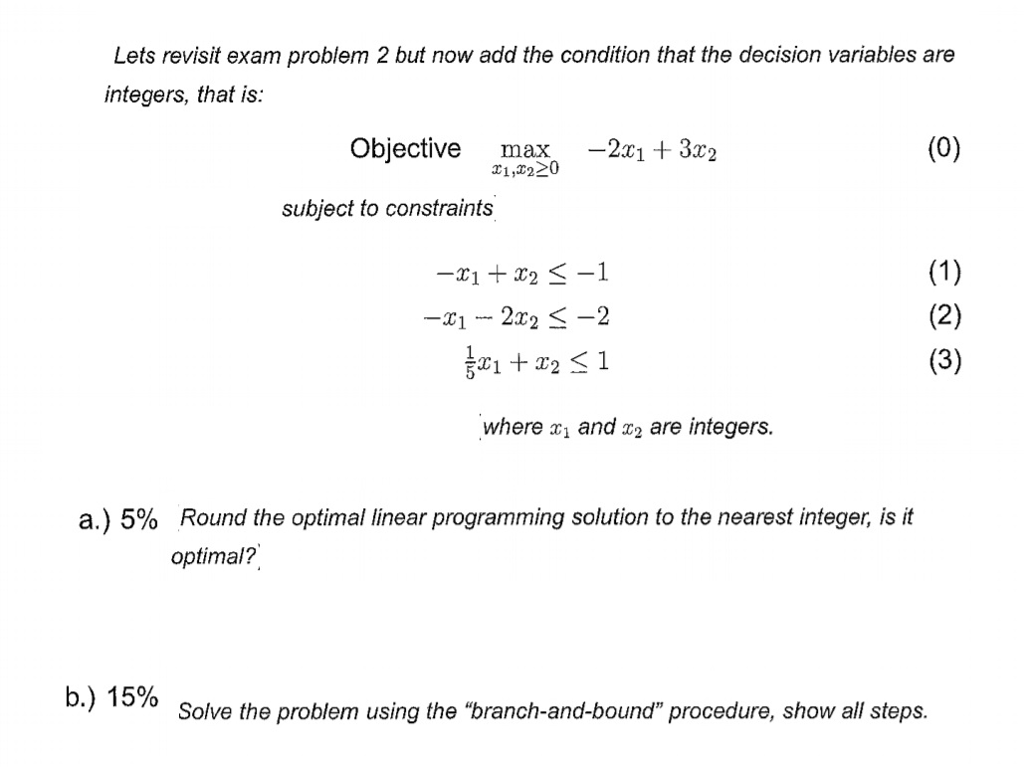Lets revisit exam problem 2 but now add the condition that the decision variables are integers, that is: Objective max_x_1, x_2 greaterthanorequalto 0 -2x_1 + 3x_2 -x_1 + x_2 lessthanorequalto -1 -x_1 – 2x_2 lessthanorequalto -2 1/5 x_1 + x_2 lessthanorequalto 1 where x_1 and x_2 are integers. a) 5% Round the optimal linear programming solution to the nearest integer, is it optimal? b.) 15% Solve the problem using the “branch-and-bound” procedure, show all steps.